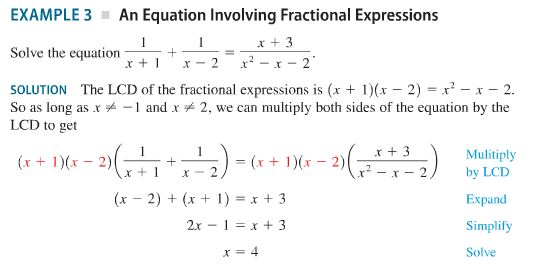EXAMPLE 3 An Equation Involving Fractional ExpressionsSolve the equation--+SOLUTION The LCD of the fractional expressions is (x + 1)(x-2) x2-x-2.LCD to get+3 Mulitiply2) by LCTx+x-2ExpandSimplifySolve

Question

This example problem has me so confused. It has been so long since I have taken Algebra. Please help me understand how they found the LCD, multiplied by the LCD, expanded, and simplified this problem. I have attached the photo of the example. Thank you!help_outlineImage TranscriptioncloseEXAMPLE 3 An Equation Involving Fractional Expressions Solve the equation--+ SOLUTION The LCD of the fractional expressions is (x + 1)(x-2) x2-x-2. LCD to get +3 Mulitiply 2) by LCT x+x-2 Expand Simplify Solve fullscreen
Step 1

The Lowest Common Denominator(LCD) is found be multiplying the denominator terms of the two fractions on the left hand side,namely, (x+1) and (x-2).

Step 2

Multiplying both sides of the equat...

Want to see the full answer?

See Solution

Want to see this answer and more?

Our solutions are written by experts, many with advanced degrees, and available 24/7

See Solution
Tagged in

Algebra